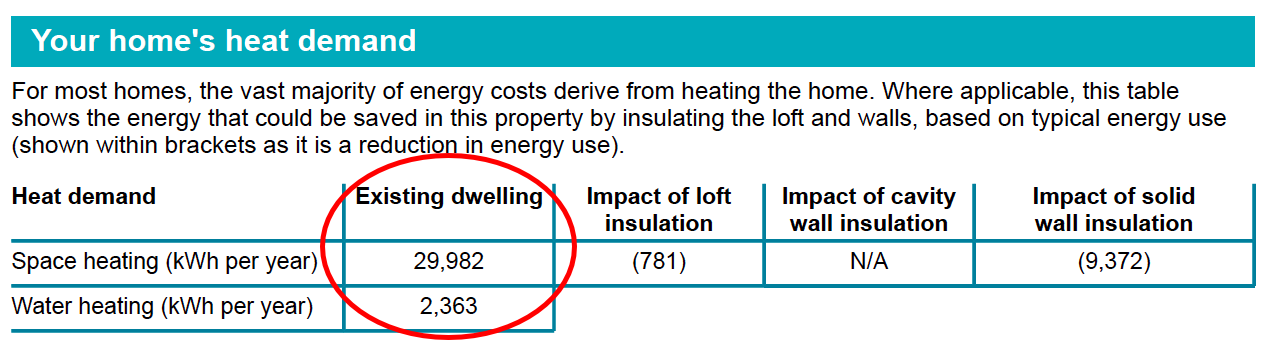At EPC London, we carry out hundreds of EPC assessments every week across London and the surrounding counties. Now despite requiring a Green Deal Report to actually apply for the Renewable heat incentive, the actual RHI payment is based on the heat requirement that comes from the EPC – so in the article below we are going to explain how you (armed with your EPC) can calculate your RHI payment and see if making the investment in renewable heating systems may be worth doing.

## How to calculate the RHI payment from your EPC

At the bottom of page 4 of the EPC is a small table that provides your home’s heat demand; we have included an example from an EPC we have carried out recently.In the second column you will see two numbers under the title Existing dwelling – the sum of these two numbers shows the total heat demand of the property in terms of space heating (e.g. radiators) and water heating (e.g. baths and showers).

The total heat demand from this EPC is 32,345 kWh of heat.

Now in terms of calculating the RHI payments, the methodology varies depending on the technology in question. We will start with biomass boilers

## Calculating the RHI for Biomass boilers

This is probably the simplest RHI calculation, because all of the heat you produce from a biomass boiler is deemed as renewable, this is because the fuel that powers the biomass boiler is produced is renewable (compare this with a heat pump where the unit requires electricity from the grid to run).

The RHI payment for Biomass boilers is currently 12.2p per kWh per year (although DECC have the right to adjust the RHI rate every 3 months).

Therefore for this property the annual return for installing a biomass boiler would be:

32,345 x £0.122 = £3,946 per year

## Calculating the RHI for Air and Ground Source Heat Pumps

Calculating the RHI for heat pumps is slightly more complicated, although the basis behind the calculation is the same for both air source and ground source heat pumps.

The reason for the complexity is the fact that the heat pump units run on electricity from the grid, so the Government will only pay the household for the units of ‘renewable heat’ produced – this is referred to as the Eligible Heat Demand.

The Eligible Heat Demand is calculated as the total annual energy demand from the EPC x (1 – 1 / SPF).

The SPF in this calculation is the seasonal performance factor and tells you how efficient the unit is. For example an electric radiator has an SPF of 1 – which means that one unit of electricity is turned into one unit of useful heat.

The SPF of a heat pump can vary from 2.5 to 5.5 (for some ground source heat pumps). The bigger the SPF the better – because this means that more renewable heat is being produced from each unit of electricity required to operate the heat pump.

So for example – imagine we were installing a ground source heat pump in the property above where the SPF is 4.5 then the calculation would be:

32,345 x (1 – 1 / 4.5) = 25,157 kWh of eligible heat under the RHI scheme

This number is then simply multiplied by the RHI for Ground source heat pumps which is currently 18.8p / kWh, so this would give an annual return of £4,730 per year guaranteed for 7 years.

Compare this for an air source heat pump where the SPF might be just 3

32,345 x (1 – 1 / 3) = 21,563 kWh of eligible heat under the RHI scheme

This number is then multiplied by the RHI for Air source heat pumps (7.3 p / kWh) to give an annual return of £1,574.

## A couple of important points to mention about the RHI

You may be wondering why we have left out solar thermal from the info above, well the RHI for solar thermal is calculated only off the back of the MCS calculations provided by the installer. The solar thermal calculation does not take into account the EPC figure and the reason for this is pretty much down to the fact that solar thermal is not going to provide all your heat demand for the house, it is there simply as a supplementary heat demand.

In addition the SPF should be calculated by the MCS installer for all heat pump calculations. The higher the SPF calculation the better in terms of the amount paid through the RHI.

Finally the RHI payment has been designed to pretty much cover the install costs of the measures. i.e. over the 7 years, a GSHP will produce a return of roughly £20-30k, an ASHP just £7-10k.